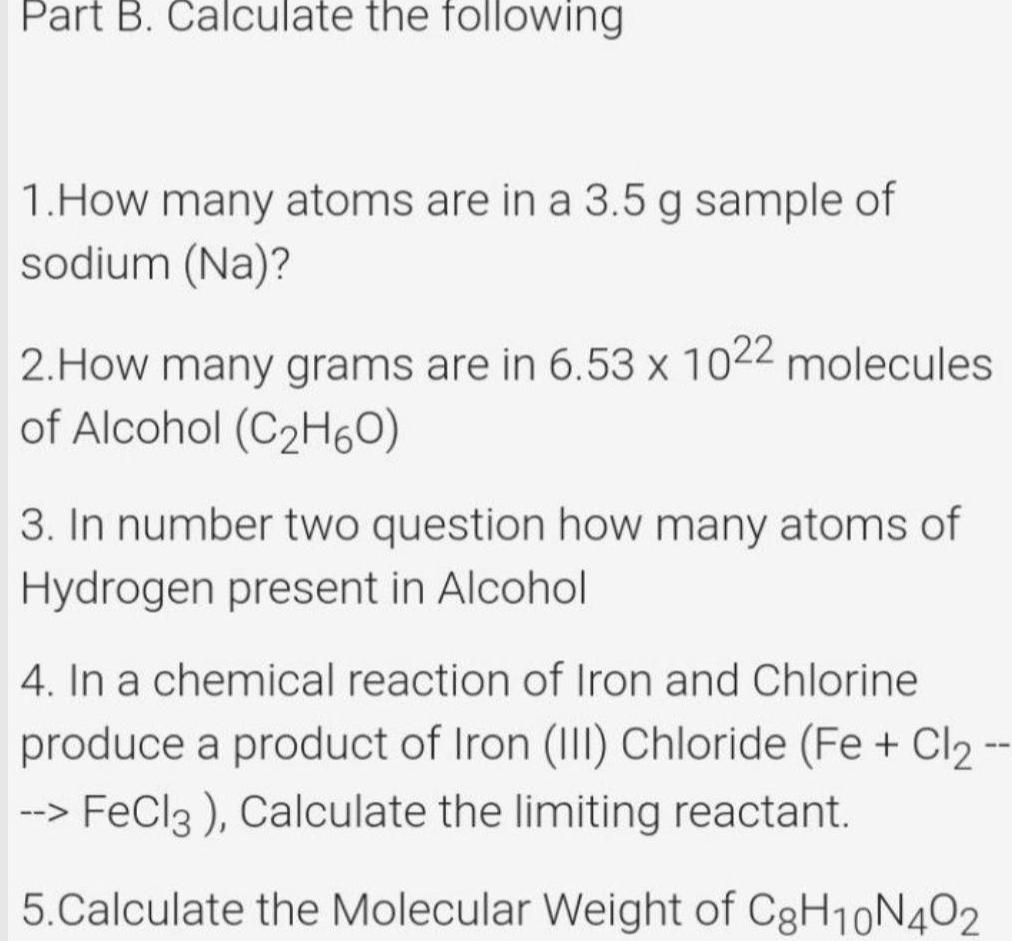Question:

# Part B Calculate the following 1 How many atoms are in a 3 5

Last updated: 7/4/2023Part B Calculate the following 1 How many atoms are in a 3 5 g sample of sodium Na 2 How many grams are in 6 53 x 1022 molecules of Alcohol C H6O 3 In number two question how many atoms of Hydrogen present in Alcohol 4 In a chemical reaction of Iron and Chlorine produce a product of Iron III Chloride Fe Cl FeCl3 Calculate the limiting reactant 5 Calculate the Molecular Weight of C8H10N 402